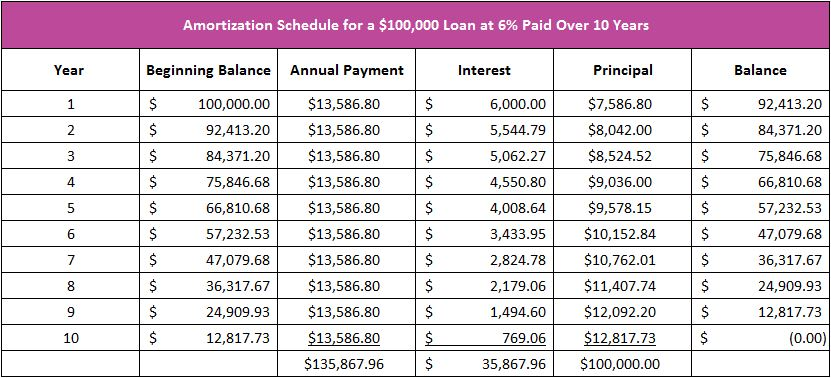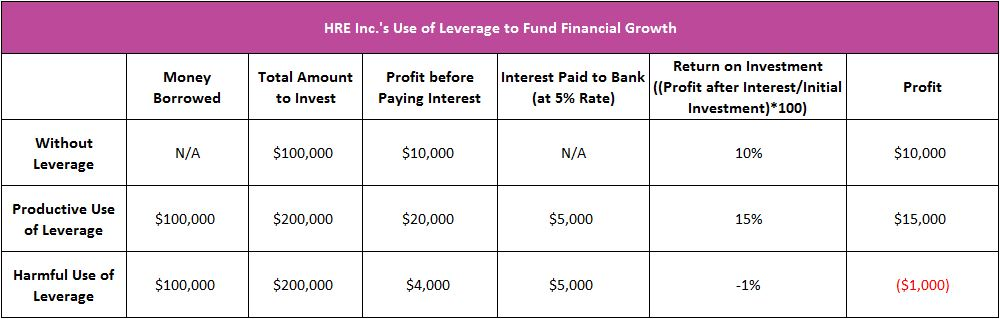# Debt

## Definition of Debt:

A debt is a loan, or money that one party borrows from another.

## Detailed Explanation:

Bank loans, bonds, credit card loans, student loans, and mortgages are examples of debt. The terms of the debt require the loan plus interest to be repaid during a specified period of time. Payments of debt include principal and interest. The principal is the amount borrowed or the balance of the amount that was originally borrowed and has not been paid off. Interest is the price of debt or the cost of borrowing.

The Amortization Schedule

A loan’s amortization schedule provides a payment's allocation to interest and principal. It is helpful in understanding how debt works and its dangers. When a payment is made, the interest is paid first. Interest is the price for the use of money, and is directly related to the loan balance and the interest rate, so the larger the loan balance or the higher the interest rate, the greater the interest expense.

The table below is the amortization schedule for a \$100,000 loan with an interest rate of six percent that is paid over a 10 year period. A \$13,586.80 payment is due annually. During the first year, \$6,000 in interest is paid (.06 x \$100,000). The principal portion of the payment is derived from subtracting the interest from the payment. In our example, the reduction of principal after the first payment equals \$7,586.80, or the difference between the \$13,586.80 payment and \$6,000 in interest. The loan balance at the end of the first year is \$92,413.20, which equals \$100,000 (the beginning balance), less \$7,586.80 (the principal paid). The amortization schedule below shows that more interest is paid in the early stages of a loan. This is because interest is paid on the outstanding balance, and the loan balance has decreased in the second year. In the second year, the interest portion of the payment equals \$5,544.80 (\$92,413.20 x .06), which means that \$8,042 is paid to the principal (\$13,586.80 - \$5,544.80).A borrower can pay off a loan sooner and save money by making payments larger than the minimum required. The higher payment negates the cost of future interest on any reduction in the loan balance. For example, the borrower would save \$6,517.10 if a \$25,000 payment is made in the second year. This is entirely due to a reduction in interest paid over the remaining term of the loan.

Business owners frequently prefer using a loan (debt) to selling stock (equity) because they do not give up an ownership interest when they borrow money. At lower borrowing rates, more projects are economically attractive, so companies can grow faster. Using debt to finance growth is an example of leverage. A business grows faster when it leverages its debt properly.

To illustrate, assume HRE, Inc. has \$100,000 to invest and projects a 10% return on its investment. This means HRE would earn a \$10,000 profit (10% of \$100,000). Management could borrow \$100,000 at a 5% rate. HRE would have \$200,000 to invest (the initial \$100,000 plus the borrowed \$100,000). HRE’s projected profit before paying interest increases to \$20,000 (10% of \$200,000). However, HRE would need to pay the bank \$5,000 for its loan (5% of \$100,000), so the projected profit after paying the loan equals \$15,000. This means HRE would earn \$15,000 after investing \$100,000 of its own funds, thus netting a 15% return on investment ((\$15,000 / \$100,000) x 100).

This is a productive use of leverage, but leverage can be risky. Remember the bank needs to be paid, so if the project fails, HRE may need to sell other assets to make its payment. For example, assume that HRE invests the \$200,000, but earns only \$4,000 before paying the bank. After paying the bank, HRE would lose \$1,000. The return on investment would drop from a positive 10% to a negative 1%. Leverage reduces a company’s profit anytime the amount earned on the borrowed funds is less than the interest paid. The table below summarizes HRE’s use of leverage.Interest rate manipulation is fundamental to monetary policy. During recessionary periods, the central bank will lower interest rates. Lower rates attract more investment, which stimulates the economy. A central bank will raise interest rates during periods when the economy is overheated and inflation becomes a great concern. Higher rates result in fewer business investments. The rate of economic growth decreases.

## Dig Deeper With These Free Lessons:

Economics – Managing Our Scarce Resources